# NSEP: How To Prepare Unit On Simple Harmonic Motion

###### BySumit Saini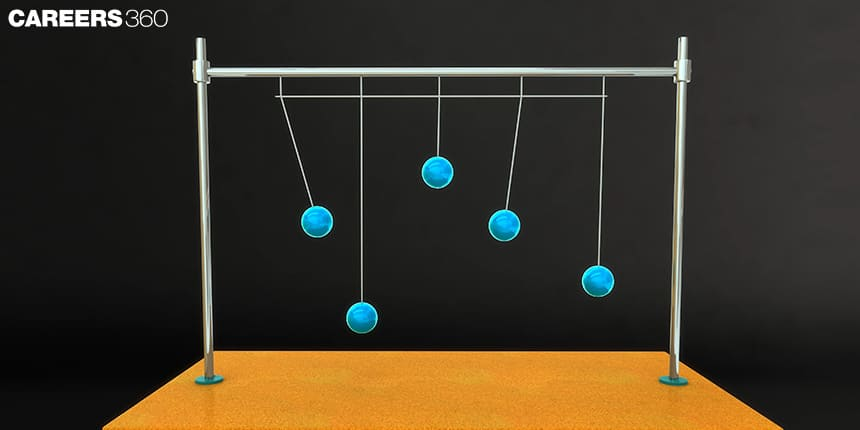###### Synopsis

Simple Harmonic Motion is one of the important topics for the National Standard Examination in Physics (NSEP). It is important to understand the basic concepts of S.H.M to solve the questions. In this article we have discussed the concepts, formulas and Previous year questions of S.H.M in NSEP. Read on to know more.###### Synopsis

Simple Harmonic Motion is one of the important topics for the National Standard Examination in Physics (NSEP). It is important to understand the basic concepts of S.H.M to solve the questions. In this article we have discussed the concepts, formulas and Previous year questions of S.H.M in NSEP. Read on to know more.

Indian Association Of Physics Teachers (IAPT) conducts the National Standard Examination In Physics (NSEP) every year which includes the syllabus of class 11 and class 12th. It is necessary to have comprehensive coverage of the syllabus to qualify for the NSEP exam. Here we discuss some of the important concepts of Simple Harmonic Motion along with Previous year questions for the NSEP examination.

NSEP Syllabus for the Simple Harmonic Motion includes Equation of simple harmonic motion( SHM), phase, oscillations of a loaded spring restoring force, Kinetic and Potential energy of the SHM system, Total energy of the SHM, derivation of the time period for simple pendulum.

Simple harmonic motion belongs to the category of periodic motion in which repetitive movement of an object takes place in a back and forth manner through an equilibrium position. In SHM, maximum displacement on one side of the equilibrium is equal to the maximum displacement on the other side. The force on the moving object in SHM is always directed towards the equilibrium position and is directly proportional to the magnitude of the object's displacement from the mean position.

Also, Read| How to Prepare Electrostatics for NSEP

Mathematically, Force in SHM is represented as:

F = −kx, where F is the force, x is the displacement, and k is a constant.

This relation is known as Hooke’s law.

In this article, we will discuss the important concepts of Simple Harmonic Motion and some of the previous year questions of NSEP for Practice. Also, Students can refer to the NCERT Class 11, Physics Chapter 14:”Oscillations” to practice more problems on Simple Harmonic Motion.

## Tips To Prepare Simple Harmonic Motion For NSEP

• Study the concepts related to the SHM in NCERT Class 12 Physics chapter Oscillations.

• Solve theoretical questions and Numericals from NCERT book and NCERT exemplar.

• Solve more questions of spring systems S.H.M related to potential energy, velocity and acceleration.

• Understand how to write and derive the SHM equations using integration methods.

• Practice SHM related questions from last five year NSEP papers and make notes of the important concepts asked in the examination.

## Important Concepts In Simple Harmonic Motion

Few Important Points for SHM:

1. SHM is a special type of oscillation along with a straight line between the two extreme points.

2. While in oscillation, there is a restoring force which is directed towards the equilibrium position.

3. Mean Position is the point at which the net force acting on the particle is Zero. Mean position in SHM is known as Stable equilibrium.

4. From the mean position, the force acting on the particle is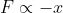; Where the negative sign represents that direction of force is opposite to

direction motion of the particle.

## Time Period and Frequency of SHM

Time Period: Time period refers to the time taken by the particle to complete one oscillation in a repeating motion. Time period in an S.H.M is represented by: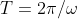Frequency: The number of oscillations completed by the object per second is known as the frequency in Simple harmonic motion.

Frequency = 1/T and, angular frequency,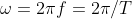## Types of Simple Harmonic Motion

Simple Harmonic Motion are of two types:

1.Linear SHM

2.Angular SHM

### Linear Simple Harmonic Motion

When a particle moves to and fro from a fixed position in a straight line in a periodic motion, it is called to be in Linear simple Harmonic Motion.

Conditions for Linear SHM:

In linear Simple harmonic Motion, the acceleration of the force acting on the particle is directly proportional to the displacement of the particle from the mean position.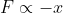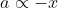• x= displacement of particle from equilibrium position

• F= Restoring force

• a= acceleration

## Angular Simple Harmonic Motion

When a particle oscillates in an angular pattern with respect to fixed axis, the particle is said to be in angular simple harmonic motion.

Conditions for Angular SHM:

The restoring torque or the Angular acceleration acting on the particle is always proportional to the angular displacement of the particle from its mean position and directed towards the equilibrium position.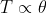and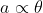Where,

• Τ =Torque

• α= angular acceleration

• θ = angular displacement

## NSEP Previous Year Questions From Simple Harmonic Motion

Q-1 (NSEP 2018-19)

After charging a capacitor C to a potential V, it is connected across an ideal inductor L. The capacitor starts discharging simple harmonically at time t = 0. The charge on the capacitor at a later time instant is q and the periodic time of simple harmonic oscillations is T. Therefore,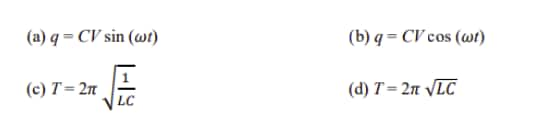Q-2 (NSEP 2017-18)

A particle rests in equilibrium under two forces of repulsion whose centers are at distances of a and b from the particle. The forces vary as the cube of the distance. The forces per unit mass are k and kʹ respectively. If the particle be slightly displaced towards one of them the motion is simple harmonic with the time period equal to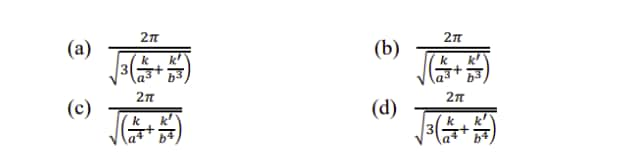Q-3 (NSEP 2015-16)

A particle executes a periodic motion according to the relation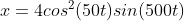.Therefore, the motion can be considered to be the superposition of n independent simple harmonic motions, where n is

(a) 2 (b) 3 (c) 4 (d) 5

Q-4 (NSEP 2016-17)

The variation of acceleration with time for a particle performing simple harmonic motion along a straight line is as in the adjacent figure.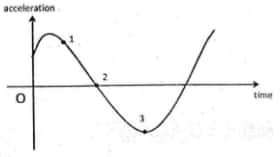Therefore,

(A) the particle has non – zero displacement initially.

(B) the displacement of the particle at point 1 is negative

(C) the velocity of the particle at point 2 is positive.

(D) the potential energy at point 3 is maximum

Also, Read| How To Prepare Magnetic Effects Of Current

## NSEP Study Materials For Simple Harmonic Motion

• NCERT Class 11 Physics chapter Oscillations

• NCERT Exemplar problems from Oscillations

• NSEP previous year's question papers

• NSEP previous year questions that are available at official website of IAPT https://iapt.org.in

Difficulty level of the NSEP questions is higher than the CBSE and JEE Mains level. Good conceptual understanding along with problem solving skills is a must to ace the NSEP exam.

• Nature
• Physical sciences
• Quantity
• Physics
• Applied and interdisciplinary physics
• Physical quantities
• Force
• Classical mechanics
• Mechanics
• Metrology

## Careers360 helping shape your Career for a better tomorrow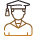#### 250M+

Students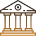#### 30,000+

Colleges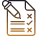#### 500+

Exams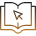E-Books